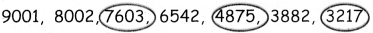Students can download 4th Maths Term 1 Chapter 2 Numbers Ex 2.2 Questions and Answers, Notes, Samacheer Kalvi 4th Maths Guide Pdf helps you to revise the complete Tamilnadu State Board New Syllabus, helps students complete homework assignments and to score high marks in board exams.

## Tamilnadu Samacheer Kalvi 4th Maths Solutions Term 1 Chapter 2 Numbers Ex 2.2

Question 1.
Circle the odd numbers in the following.
9001, 8002, 7603, 6542, 4875, 3882, 3217.Question 2.
Circle the even numbers in the following.
6231,5920, 4812, 2121, 1234, 9528, 3946.Question 3.
Choose the even numbers from the following and write the number and number names.
a. 6501
b. 4706
c. 3999
d. 4001
e. 3848

 Number Number Name b. 4706 Four thousand seven hundred and six. e. 3848 Three thousand eight hundred and Forty eight.Question 4.
Pick out the even numbers and write their name in the table given below,
a. 4703
b. 3206
c. 2003
d. 4017
e. 2001